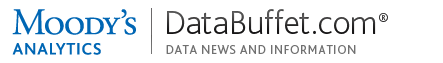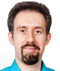Logout / Access Other productsGet live help Monday-Friday from 7:00AM-6:00PM ET (11:00AM-10:00PM GMT)  •  Contact Us
Check out our new FAQ section!Using Data Buffet: TransformationsMonday, 26 Oct 2015 14:39 ETBy Phillip Thorne
 Summary DataBuffet.com provides mathematical "transformations" to examine level and rate changes in time series. This article explains how each one is computed and invoked. Detail Introduction The transformations are available in View, Basket and Chart modes, and in the Moody's Analytics Power Tools add-in for Microsoft® Excel. If you need to duplicate these computations outside Moody's Analytics software, implement the mathematical formulas shown below. Transformations are distinct from the Data Buffet frequency conversions feature.  They are agnostic as to index base year, currency base year (nominal/real), seasonal adjustment, and all other economically meaningful properties. All of the "canned" transformations in the web interface, plus others, are available as functions that can be used in Data Buffet basket formulas. You can insert a formula wherever you'd use a single mnemonic, either in the Grid Editor or Power Editor. An outermost pair of parentheses is required, which signals the DataBuffet.com engine that a formula is present. Formulas are useful when you need custom processing beyond the canned transformations, but they can be tricky to debug; rather than jumping directly to a complex formula, test that each of the operands and sub-expression works correctly. Data Buffet formula syntax X[t]   = The value of time series X at period t X[t-1] = The value of time series X at one period before t p      = Periodicity, the number of periods per year. For annual, quarterly, monthly, and weekly series, p =1,  4, 12, and 52, respectively. **     = Exponentiation 1. Simple difference The difference in level between two consecutive periods, expressed in the same units as the operands. Appears on DataBuffet.com as: "Simple Difference" (X[t] - X[t-1]) Basket function: (DIFF(X)) 2. Year-over-year difference The difference in level between nonconsecutive periods one year apart.  Same as simple difference if the frequency is annual (p=1). Appears as: "Year over Year Difference" (X[t] - X[t-p]) Basket function, monthly series: (DIFF(X, 12)) Basket function, quarterly series: (DIFF(X, 4)) Basket function with a 3-period lead: (DIFF(X, -3)) 3. Percent change The percent change between consecutive periods. Appears as: "% Change" (((X[t] - X[t-1]) / X[t-1]) * 100) Example: ((Income[Apr] - Income[Mar]) / Income[Mar]) * 100 Basket function: (PCH(X)) 4. Year-over-year percent change The percent change between nonconsecutive periods one year apart.  Same as percent change if the frequency is annual (p=1). Appears as: "Year over Year %" (((X[t] - X[t-p]) / X[t-p]) * 100) Basket function: (PCHY(X)) Note: This formula can be adapted to compute the change over an arbitrary period. For example, for a three-year change in a quarterly series, use p=12. 5. Annualized growth rate The growth rate between consecutive periods, annualized; expressed in percent. Appears as: "Annualized Growth" ((((X[t] / X[t-1])**p)-1) * 100) Example: ((((Population[08Q2] / Population[08Q1]) ^ 4) - 1) * 100) Basket function: (PCHA(X)) 6. Uncentered moving average This does not appear in the interface, but is available as a basket function. Using the default number of periods (12 for a monthly series, 4 for quarterly): (MAVE(X)) Three-period moving average: (MAVE(X, 3)) 7. Compound annualized growth rate DataBuffet.com does not have a interface transformation or basket function for CAGR.  However, you can build a custom basket formula with arithmetic and date index operators.  For more info on CAGR, please see Wikipedia or the financial-math reference of your choice. A time series of the CAGRs of a 10-year T-note (monthly series) over 3 years (36 months): ((IRGT10YM.US[T] / IRGT10YM.US[T-36])**(1/3) - 1) Over a 5-year (72-month) horizon, values expressed in percent: (((IRGT10YM.US[T] / IRGT10YM.US[T-72])**(1/5) - 1) * 100) Other